# CCJ’s Coding Note 2021 (1) - Basic Knowledge Points Summary

Written by CCJ, Monday, Jan 18, 2021 @ Secaucus, NJ, USA.

## DFS - Path Finding

• find a path between two given vertices u an z;

• Use a stack S to keep track of the path between the start vertex and the current vertex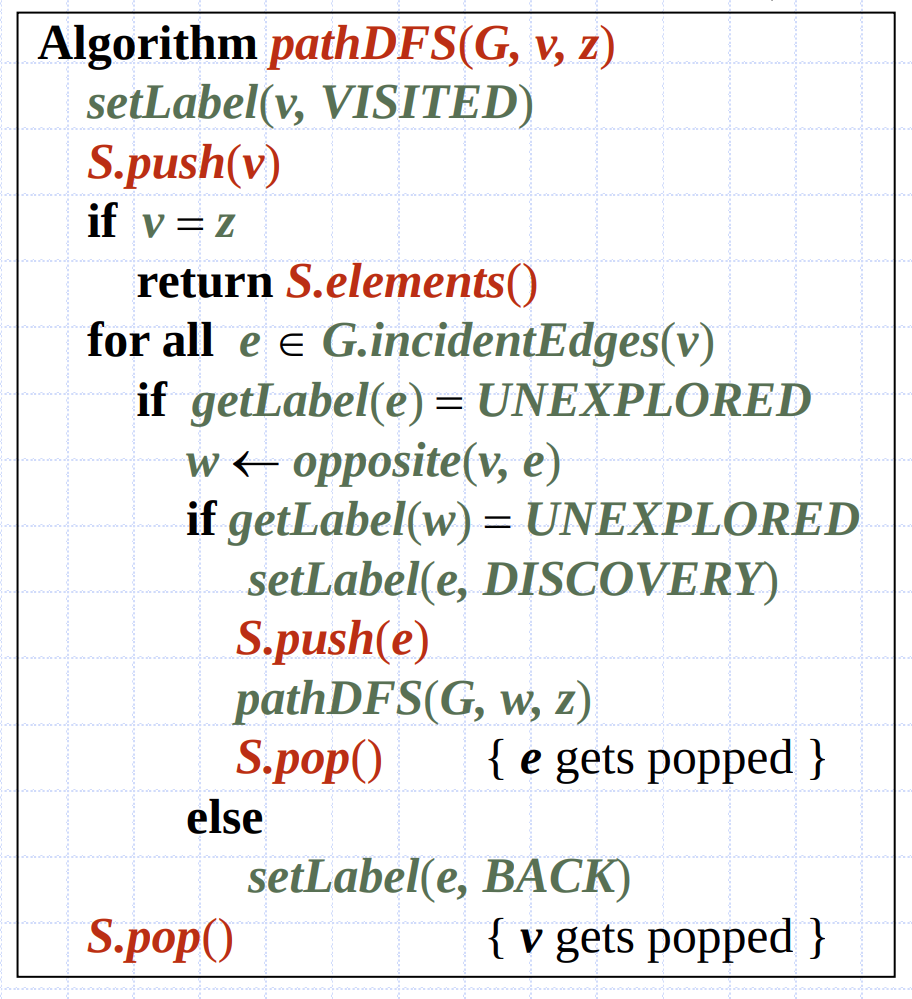## DFS - Cycle Finding

• use a stack S to keep track of the path between the start vertex and the current vertex

• As soon as a back edge (v, w) is encountered, we return the cycle as the protion of the stack from the top to vertex 𝑤w

• Shown as below: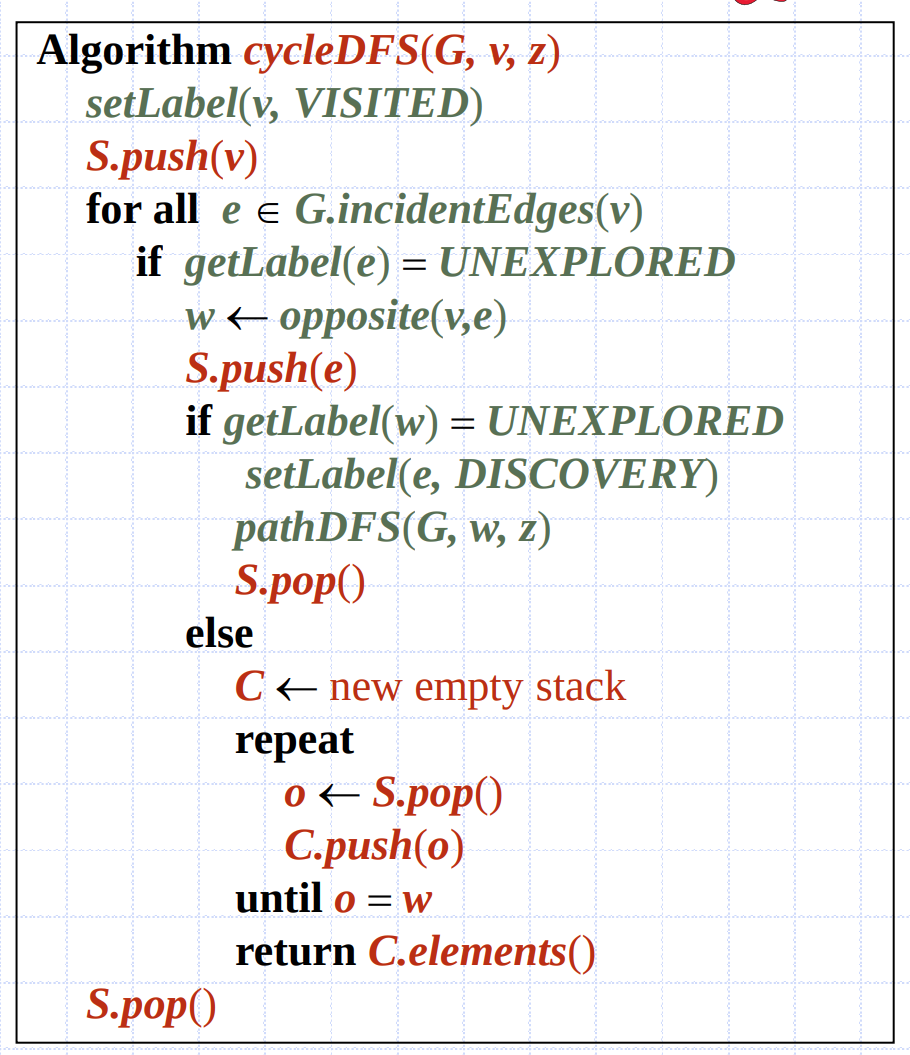## Graph - Adjacency List Representation

• Graph in Python can be implemented by defaultdict;

• DFS and BFS are implemented below;

# Python3 program to print DFS traversal
# from a given given graph
from collections import defaultdict

# This class represents a directed graph using
class Graph:

# Constructor
def __init__(self):
# default dictionary to store graph
# Using List as defaultdict's default_factory
self.graph = defaultdict(list)

# function to add an edge to graph
self.graph[u].append(v)

# A recursive function used by DFS
def DFSUtil(self, v, visited):
# Mark the current node as visited and print it
visited[v] = True
print(v, end = ' ')

# Recur for all the vertices adjacent to this vertex
for i in self.graph[v]:
if visited[i] == False:
self.DFSUtil(i, visited)

# The function to do DFS traversal. It uses
# recursive DFSUtil()
def DFS(self, v):
# Mark all the vertices as not visited
visited = [False] * (max(self.graph)+1)

# Call the recursive helper function to print DFS traversal
self.DFSUtil(v, visited)

# Function to print a BFS of graph
def BFS(self, s): # s is int value;
# Mark all the vertices as not visited
visited = [False] * (len(self.graph))

# Create a queue for BFS
queue = []

# Mark the source node as visited and enqueue it
queue.append(s)
visited[s] = True

while queue:
# Dequeue a vertex from queue and print it
# current vertex v:
# and the first value of v will be the starting node s;
v = queue.pop(0)
print (v, end = " ")

# Get all adjacent vertices of the
# dequeued vertex v. If an adjacent
# has not been visited, then mark it
# visited and enqueue it
for i in self.graph[v]: # for all incidentEdges(v), that is,edge (v, i)
if visited[i] == False:
queue.append(i)
visited[i] = True


## Heap and Heapify

### 1) Definition

A heap is a binary tree storing keys at its internal nodes and satisfying the following properties:

• Heap-Order: for every internal node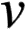other than the root, key()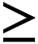key(parent()).

• Complete Binary Tree: let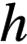be the height of the heap

• for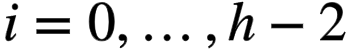, there are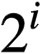nodes of depth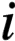;

• at depth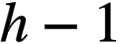, the internal nodes are to the left of the external nodes. By saying that all the internal nodes on levelare “to the left” of the external nodes, we mean that all the internal nodes on this level will be visited before any external nodes on this level in an inorder traversal.

The last node of a heap is the rightmost internal node of depth h − 1.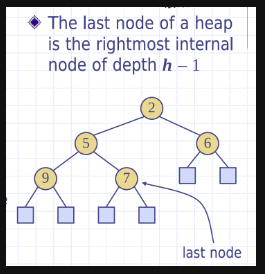### 2) Height of a Heap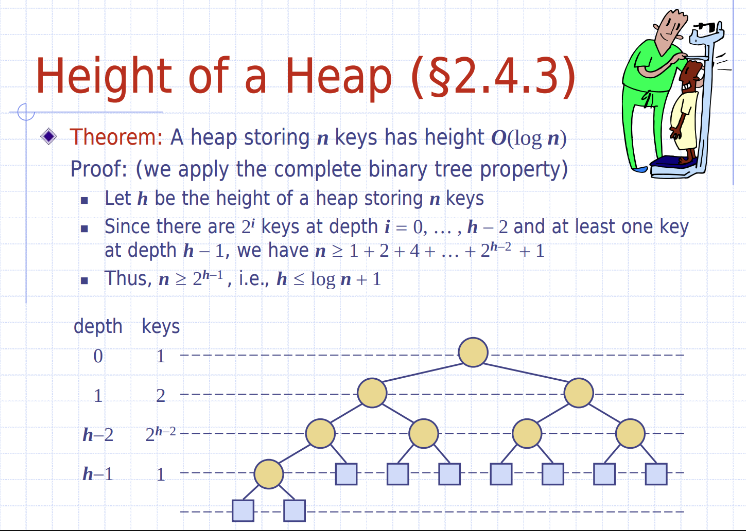### 3) Insertion into a Heap and Up-heap Bubbling

• Method insertItem(k): insert a key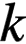to the heap.